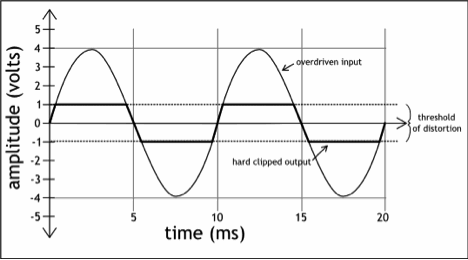# Square Wave Calculations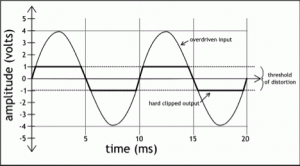Hard clip a sine wave and it becomes square-ish, very square-ish.

This suggests one way to learn to hear distortion:  listen for the sound of the associated harmonic structure.

What are the harmonics in a square wave?  Glad you asked.  An equation can spell it out precisely.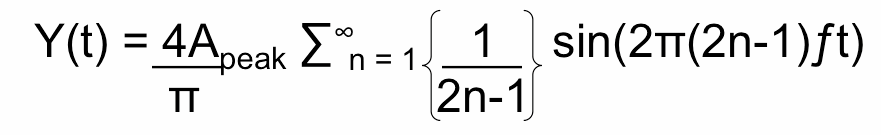Where Apeak is the peak amplitude of the square wave, ƒis frequency in Hertz, and t is time in seconds.

Let’s put the equation to work.  We’ll set the peak amplitude to 1 volt, and step through the first three harmonics by letting n = 1, 2, and then 3.  Let’s build a square wave with a fundamental frequency of 100 Hz.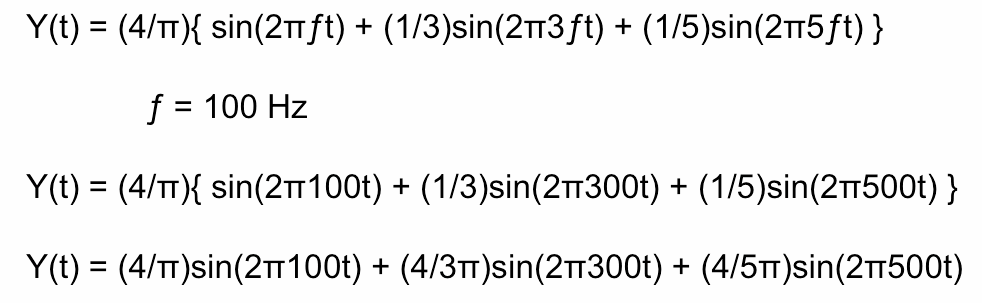Let a computer crunch the numbers and we can begin to graph the square wave.  The image on the left is a plot in the time domain – the  amplitude versus time waveform you see in your digital audio workstation.  The image on the right is the all-important frequency representation of the same wave.  It shows the amplitude as a function of frequency.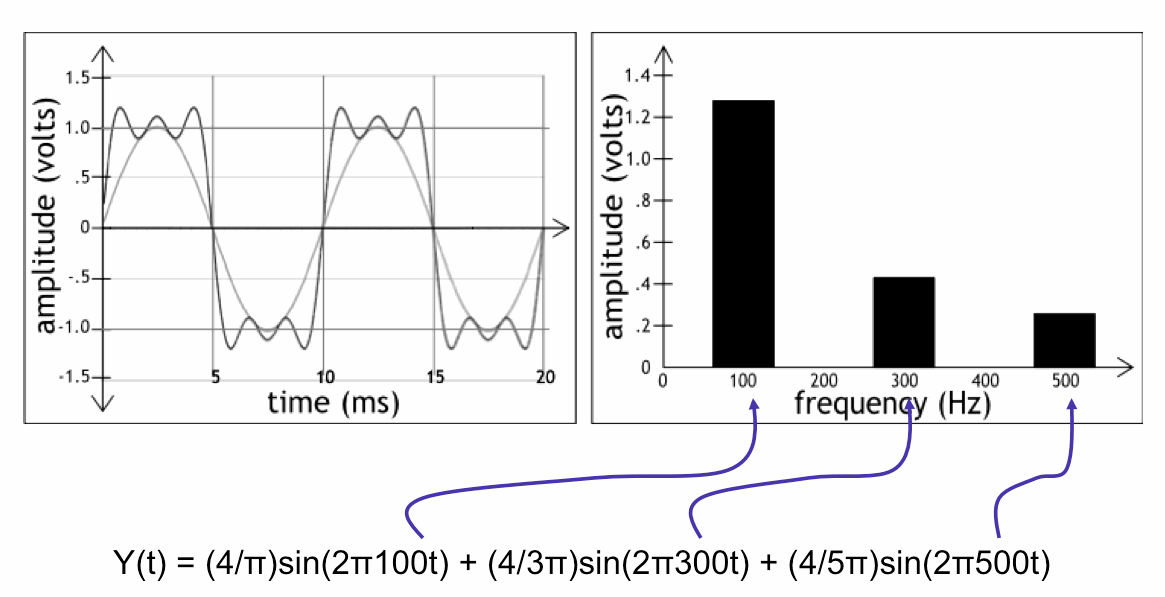Three harmonics – those three spikes on the frequency domain plot – add up to a bat-signal sort of wave form in the time domain.  It’s not yet square, because we need more harmonics.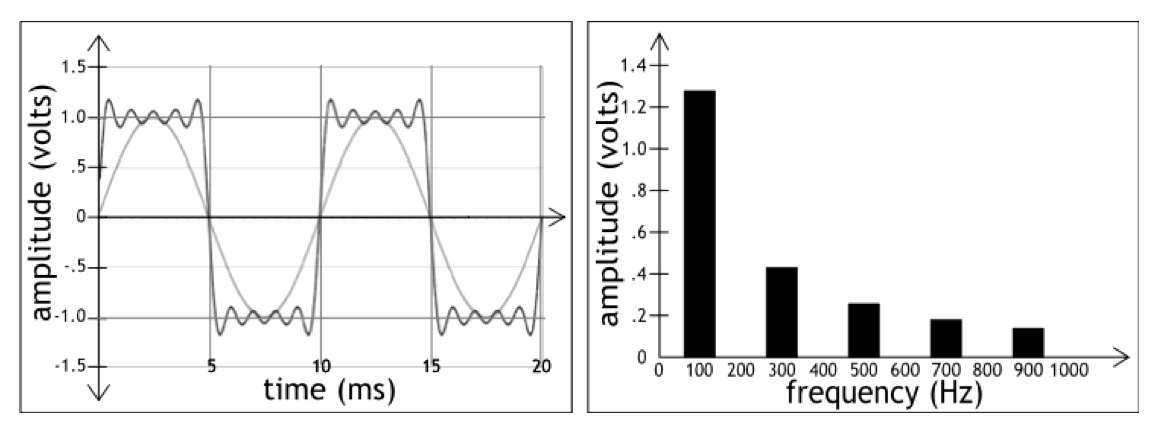With five harmonics, a crude square wave form takes shape.

Crunching thirteen harmonics gets us closer.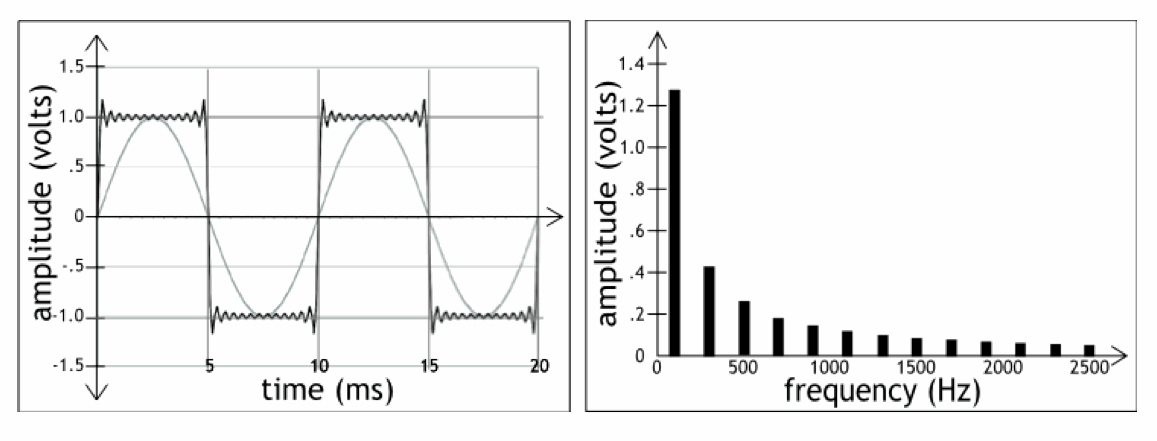Including twenty-five harmonic components, closer still.  In all cases, we carefully follow the equation above, choosing the right frequency components, each with exactly the right amplitude needed to make a square wave.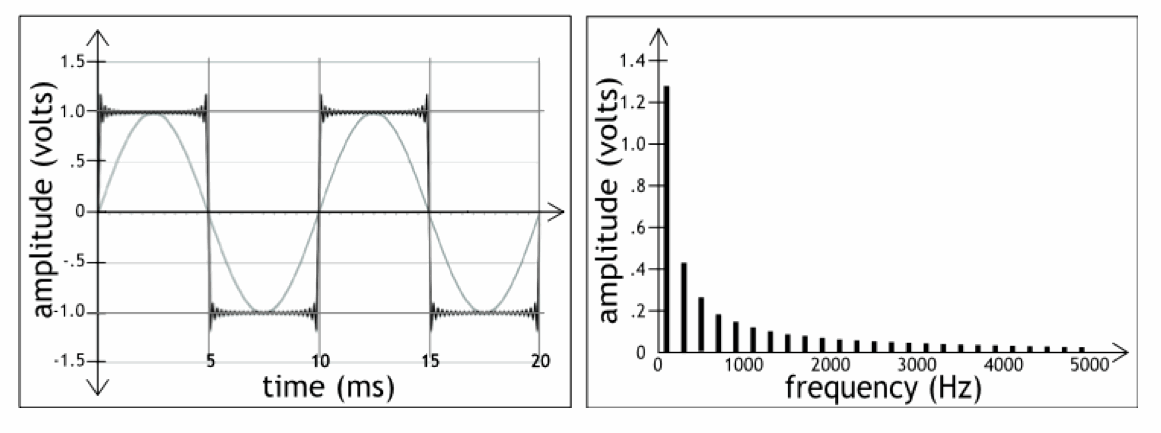This specific recipe of odd harmonics will create a square wave that is perfectly square, if you have a bandwidth that goes to, um, infinity.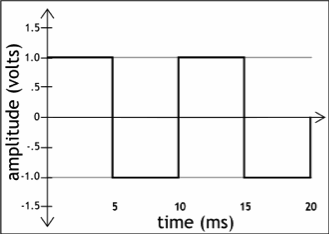As the bandwidth narrows, as it rolls of the high frequencies, the wave becomes less square.

Learn the sonic signature of this edgy recipe of harmonics, and you’ll learn to spot distortion when things are going wrong, and you’ll have an aural starting point for when you choose to distort on purpose.

Afterall, the only difference between this

440 Hertz Sine Wave 44o Hz Sine Wave

and this

440 Hard Clip Clipped 44o Hz Sine Wave

is the harmonics.

This video illustrates the point: